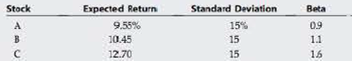Chapter 8, Problem 13PFundamentals of Financial Manageme...

15th Edition
Eugene F. Brigham + 1 other
ISBN: 9781337395250

Solutions

Chapter
SectionFundamentals of Financial Manageme...

15th Edition
Eugene F. Brigham + 1 other
ISBN: 9781337395250
Textbook Problem

CAPM, PORTFOLIO RISK, AND RETURN Consider the following information for Stocks A, B, and C. The returns on the three stocks are positively correlated, but they are net perfectly correlated. (That is, each of the correlation coefficients is between 0 and 1.)Fund P has one-third of its funds invested in each of the three stocks. The risk-free rate is 53%, and the market is in equilibrium. (That is, required returns equal expected returns.)a. What is the market risk premium (rM – rRF)? b. What is the beta of Fund P? c. What is the required return of Fund P? d. Would you expect the standard deviation of Fund P to be less than 15%, equal to 15%, or greater than 15%? Explain.

a.

Summary Introduction

To determine: The market risk premium.

The Required Rate of Return:

The required rate of return is the rate which should be earned on an investment to keep that investment running in the market. When the required return is earned only then the users and the companies invest in that particular investment.

The amount of returns that exceeds the risk-free rate on an investment is known as market risk premium. The security market line’s slope represents the market risk premium.

Explanation

For stock A:

Given,

The risk-free rate is 5.5%.

The expected return is 9.55%.

The value of beta is 0.9.

The formula to calculate the market risk premium is,

(rMrRF)=rirRFbi

Where,

• ri is the required return on the stock.
• rRF is the risk-free return.
• rM is the market return.
• bi is the value of the stock’s beta.

Substitute 5.5% for rRF , 9.55% for ri , and 0.9 for bi in the above formula.

(rMrRF)=9.55%5.5%0.9=4.050.9=4.5%

The market risk premium for stock A is 4.5%.

For stock B:

The risk-free rate is 5.5%.

The expected return is 10.45%.

The value of beta is 1.1.

The formula to calculate the market risk premium is,

(rMrRF)=rirRFbi

Where,

• ri is the required return on the stock.
• rRF is the risk-free return.
• rM is the market return.
• bi is the value of the stock’s beta

b.

Summary Introduction

To determine: The beta of Fund P.

Portfolio beta:

The portfolio beta is a measure that reflects the relativity to market. It measures how the stock moves in the market. A high portfolio shows that securities are more volatile in the price movements while a low beta represents that securities are less volatile in the price movements.

c.

Summary Introduction

To determine: The required return of Fund P.

d.

Summary Introduction

To explain: The expected value of standard deviation of Fund P.

Standard deviation:

The standard deviation refers to the stand-alone risk associated with the securities. It measures how much a data is dispersed with its standard value. The Greek letter sigma represents the standard deviation.

Still sussing out bartleby?

Check out a sample textbook solution.

See a sample solution

The Solution to Your Study Problems

Bartleby provides explanations to thousands of textbook problems written by our experts, many with advanced degrees!

Get Started

Why do economists make assumptions?

Brief Principles of Macroeconomics (MindTap Course List)

Explain why economists usually oppose controls on prices.

Principles of Macroeconomics (MindTap Course List)

RATIO ANALYSIS The Corrigan Corporation's 2013 and 2014 financial statements follow, along with some industry a...

Fundamentals of Financial Management, Concise Edition (with Thomson ONE - Business School Edition, 1 term (6 months) Printed Access Card) (MindTap Course List)

LO3 To credit an account is to enter an amount on the right side of the account.

College Accounting, Chapters 1-27 (New in Accounting from Heintz and Parry)